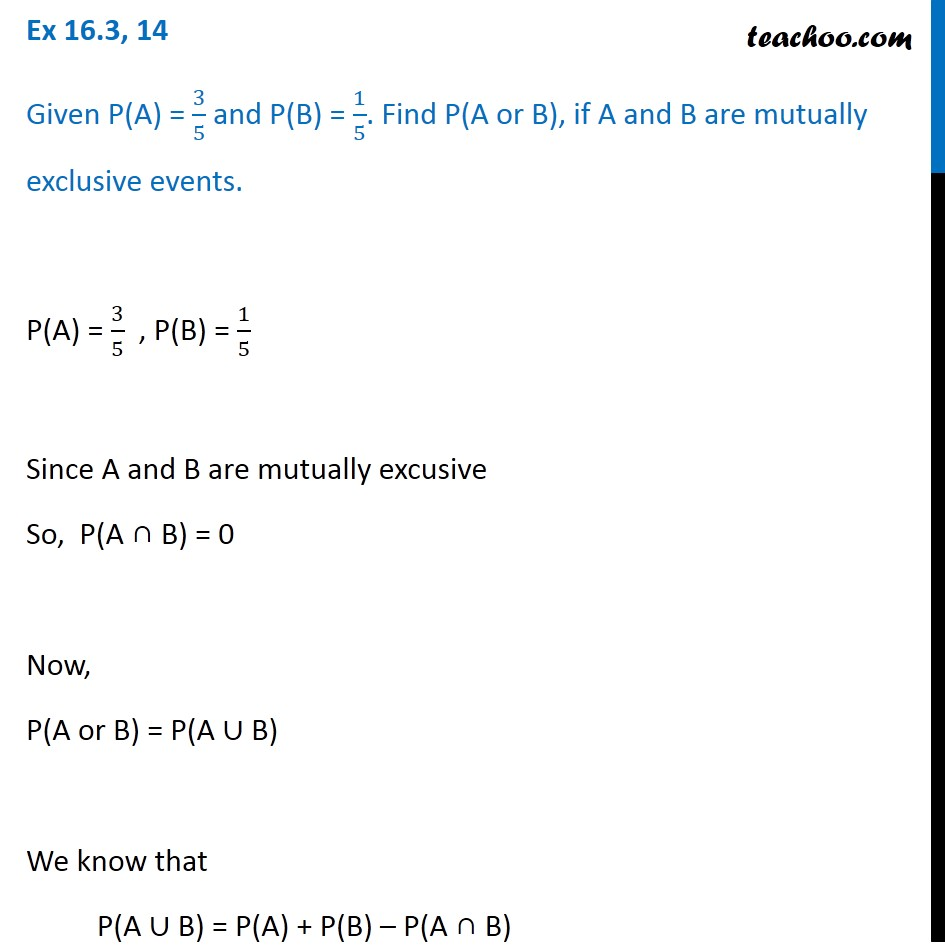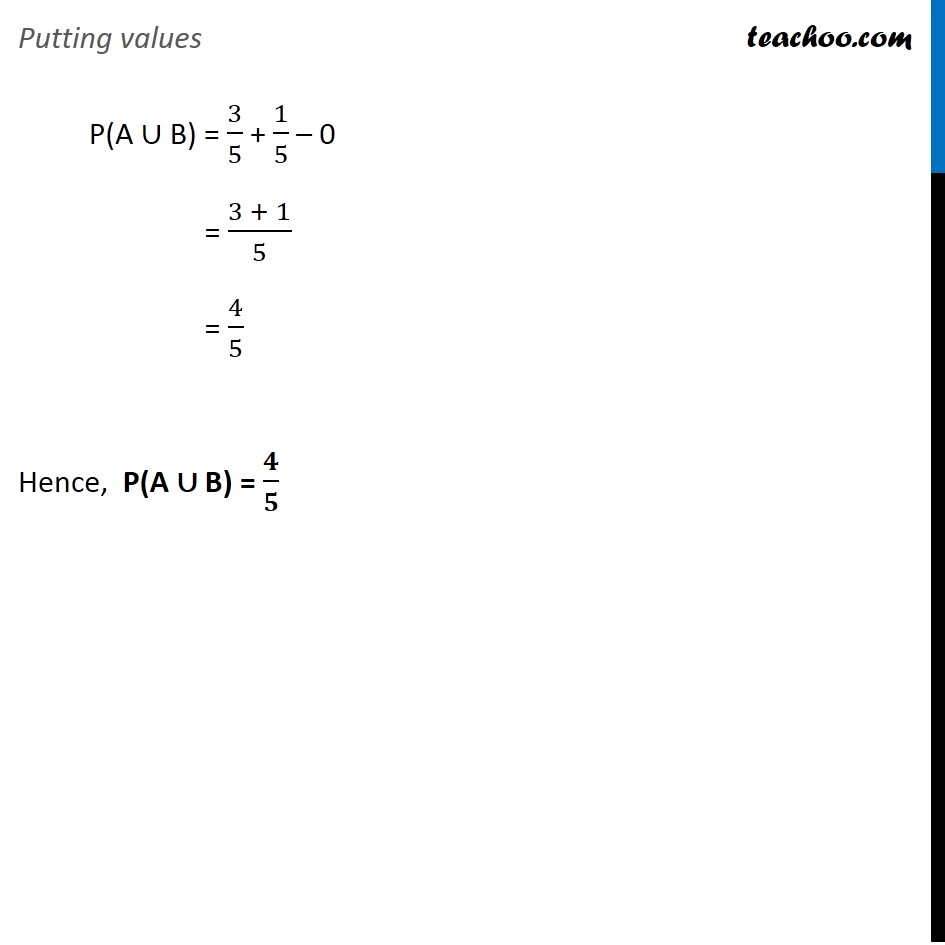Ex 14.2

Chapter 14 Class 11 Probability
Serial order wiseLearn in your speed, with individual attention - Teachoo Maths 1-on-1 Class

### Transcript

Ex 14.2, 14 Given P(A) = 3/5 and P(B) = 1/5. Find P(A or B), if A and B are mutually exclusive events. P(A) = 3/5 , P(B) = 1/5 Since A and B are mutually excusive So, P(A ∩ B) = 0 Now, P(A or B) = P(A ∪ B) We know that P(A ∪ B) = P(A) + P(B) – P(A ∩ B) Putting values P(A ∪ B) = 3/5 + 1/5 – 0 = (3 + 1)/5 = 4/5 Hence, P(A ∪ B) = 𝟒/𝟓